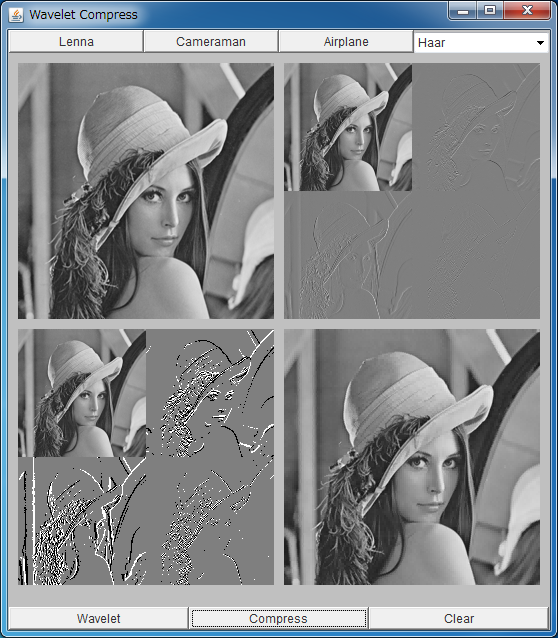# Image Compression using the Wavelet DecompositionIf the above application does not start, please install Java from www.java.com.

This applet demonstrates the image compression with the wavelet decomposition.
The so-called JPEG2000 is based on this method.

As shown in the page of "edge detection with the wavelet decomposition", the original image is reconstructed from the low-freq. and high-freq. components with the inverse wavelet transformation.

Typically, the high-freq components (or, wavelet coefficients) distribute around 0 according to the bell-shaped distribution like Gaussian.
Lets denote the standard deviation of the distribution as σ. By discarding small wavelet coefficients, the amount of the data can be reduced.

The lower-left image shows points whose wavelet coefficients are larger than σ.
By reconstructing the image with only large wavelet coefficients, the compressed image is obtained.
When the high-level wavelet decomposition is applied, the quality of the compressed image is low because the data are too much reduced.

<< Edge Detection with Wavelet

Back to Takashi Kanamaru's Web Page